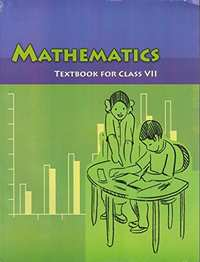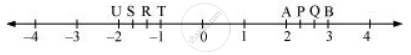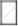Share

# NCERT solutions for Class 7 Maths chapter 9 - Rational Numbers [Latest edition]

Course
Textbook page

#### Chapters## Chapter 9: Rational Numbers

Ex. 9.1Ex. 9.2

#### NCERT solutions for Class 7 Maths Chapter 9 Rational Numbers Exercise 9.1 [Pages 182 - 184]

Ex. 9.1 | Q 1.1 | Page 182

List five rational numbers between − 1 and 0

Ex. 9.1 | Q 1.2 | Page 182

List five rational numbers between  − 2 and − 1

−2 and −1

Ex. 9.1 | Q 1.3 | Page 182

List five rational numbers between (-4)/5 and (-2)/3

Ex. 9.1 | Q 1.4 | Page 182

List five rational numbers between 1/2 and 2/3

Ex. 9.1 | Q 2.1 | Page 182

Write four more rational numbers in the following patterns:

(-3)/5, (-6)/10,(-9)/15, (-12)/20, ...

Ex. 9.1 | Q 2.2 | Page 182

Write four more rational numbers in the following patterns:

(-1)/4, (-2)/8,(-3)/12 ....

Ex. 9.1 | Q 2.3 | Page 182

Write four more rational numbers in the following patterns:

(-1)/6, 2/(-12), 3/(-18), 4/(-24)

Ex. 9.1 | Q 2.4 | Page 182

Write four more rational numbers in the following patterns:

(-2)/3, 2/(-3),4/(-6), 6/(-9)...

Ex. 9.1 | Q 3.1 | Page 183

Give four rational numbers equivalent to (-2)/7

Ex. 9.1 | Q 3.2 | Page 183

Give four rational numbers equivalent to 5/(-3)

Ex. 9.1 | Q 3.3 | Page 183

Give four rational numbers equivalent to: 4/9

Ex. 9.1 | Q 4.1 | Page 183

Draw the number line and represent the following rational numbers on it:

3/4

Ex. 9.1 | Q 4.2 | Page 183

Draw the number line and represent the following rational numbers on it:

(-5)/8

Ex. 9.1 | Q 4.3 | Page 183

Draw the number line and represent the following rational numbers on it]

(-7)/4

Ex. 9.1 | Q 4.4 | Page 183

Draw the number line and represent the following rational numbers on it:

7/8

Ex. 9.1 | Q 5 | Page 183

The points P, Q, R, S, T, U, A and B on the number line are such that,

TR = RS = SU and AP = PQ = QB. Name the rational numbers represented by P, Q, R and S.Ex. 9.1 | Q 6.1 | Page 183

Which of the following pairs represent the same rational number?

(-7)/21 and 3/9

Ex. 9.1 | Q 6.2 | Page 183

Which of the following pairs represent the same rational number?

(-16)/20 and 20/(-25)

Ex. 9.1 | Q 6.3 | Page 183

Which of the following pairs represent the same rational number?

(-2)/(-3) and 2/3

Ex. 9.1 | Q 6.4 | Page 183

Which of the following pairs represent the same rational number?

(-3)/5 and (-12)/20

Ex. 9.1 | Q 6.5 | Page 183

Which of the following pairs represent the same rational number?

8/(-5) and (-24)/15

Ex. 9.1 | Q 6.6 | Page 183

Which of the following pairs represent the same rational number?

1/3 and (-1)/9

Ex. 9.1 | Q 6.7 | Page 183

Which of the following pairs represent the same rational number?

(-5)/(-9) and 5/(-9)

Ex. 9.1 | Q 7.1 | Page 183

Rewrite the given rational numbers in the simplest form:

(-8)/6

Ex. 9.1 | Q 7.2 | Page 183

Rewrite the following rational numbers in the simplest form:

25/45

Ex. 9.1 | Q 7.3 | Page 183

Rewrite the following rational numbers in the simplest form:

(-44)/72

Ex. 9.1 | Q 7.4 | Page 183

Rewrite the following rational numbers in the simplest form:

(-8)/10

Ex. 9.1 | Q 8.1 | Page 183

Fill in the boxes with the correct symbol out of >, <, and =

(-5)/72/3

Ex. 9.1 | Q 8.2 | Page 183

Fill in the boxes with the correct symbol out of >, <, and =

(-4)/5(-5)/7

Ex. 9.1 | Q 8.3 | Page 183

Fill in the boxes with the correct symbol out of >, <, and =

(-7)/814/(-16)

Ex. 9.1 | Q 8.4 | Page 183

Fill in the boxes with the correct symbol out of >, <, and =

(-8)/5(-7)/4

Ex. 9.1 | Q 8.5 | Page 183

Fill in the boxes with the correct symbol out of >, <, and =

1/(-3)(-1)/4

Ex. 9.1 | Q 8.6 | Page 183

Fill in the boxes with the correct symbol out of >, <, and =

5/(-11)(-5)/11

Ex. 9.1 | Q 8.7 | Page 183

Fill in the boxes with the correct symbol out of >, <, and =

0(-7)/6

Ex. 9.1 | Q 9.1 | Page 184

Which is greater in the following?

2/3,5/2

Ex. 9.1 | Q 9.2 | Page 184

Which is greater in the following?

(-5)/6, (-4)/3

Ex. 9.1 | Q 9.3 | Page 184

Which is greater in the following?

(-3)/4,2/(-3)

Ex. 9.1 | Q 9.4 | Page 184

Which is greater in the following?

(-1)/4, 1/4

Ex. 9.1 | Q 9.5 | Page 184

Which is greater in the following?

-3 2/7, -3 4/5

Ex. 9.1 | Q 10.1 | Page 184

Write the given rational numbers in ascending order:

(-3)/5, (-2)/5, (-1)/5

Ex. 9.1 | Q 10.2 | Page 184

Write the given rational numbers in ascending order:

(-1)/3,(-2)/9,(-4)/3

Ex. 9.1 | Q 10.3 | Page 184

Write the given rational numbers in ascending order:

(-3)/7, (-3)/2, (-3)/4

#### NCERT solutions for Class 7 Maths Chapter 9 Rational Numbers Exercise 9.2 [Page 190]

Ex. 9.2 | Q 1.1 | Page 190

Find the sum 4/5 + ((-11)/4)

Ex. 9.2 | Q 1.2 | Page 190

Find the sum 5/3 + 3/5

Ex. 9.2 | Q 1.3 | Page 190

Find the sum (-9)/10 + 22/15

Ex. 9.2 | Q 1.4 | Page 190

Find the sum: (-3)/(-11) + 5/9

Ex. 9.2 | Q 1.5 | Page 190

Find the sum: (-8)/19 + (-2)/57

Ex. 9.2 | Q 1.6 | Page 190

Find the sum: (-2)/3 + 0

Ex. 9.2 | Q 1.7 | Page 190

Find the sum: -2 1/3 + 4 3/5

Ex. 9.2 | Q 2.1 | Page 190

Find 7/24 - 17/36

Ex. 9.2 | Q 2.2 | Page 190

Find 5/63 - ((-6)/21)

Ex. 9.2 | Q 2.3 | Page 190

Find (-6)/13 - ((-17)/15)

Ex. 9.2 | Q 2.4 | Page 190

Find (-3)/8 - 7/11

Ex. 9.2 | Q 2.5 | Page 190

Find -2 1/9 - 6

Ex. 9.2 | Q 3.1 | Page 190

Find the product: 9/2 xx ((-7)/4)

Ex. 9.2 | Q 3.2 | Page 190

Find the product: 3/10 xx (-9)

Ex. 9.2 | Q 3.3 | Page 190

Find the product: (-6)/9 xx 9/11

Ex. 9.2 | Q 3.4 | Page 190

Find the product: 3/7 xx ((-2)/5)

Ex. 9.2 | Q 3.5 | Page 190

Find the product: 3/11 xx 2/5

Ex. 9.2 | Q 3.6 | Page 190

Find the product: 3/(-5) xx (-5)/3

Ex. 9.2 | Q 4.1 | Page 190

Find the value of (-4) ÷ 2/3

Ex. 9.2 | Q 4.2 | Page 190

Find the value of: (-3)/5 ÷ 2

Ex. 9.2 | Q 4.3 | Page 190

Find the value of: (-4)/5 ÷ (-3)

Ex. 9.2 | Q 4.4 | Page 190

Find the value of: (-1)/8 ÷ 3/4

Ex. 9.2 | Q 4.5 | Page 190

Find the value of: (-2)/13 ÷ 1/7

Ex. 9.2 | Q 4.6 | Page 190

Find the value of: (-7)/12 ÷ ((-2)/13)

Ex. 9.2 | Q 4.7 | Page 190

Find the value of: 3/13 ÷ ((-4)/65)

## Chapter 9: Rational Numbers

Ex. 9.1Ex. 9.2## NCERT solutions for Class 7 Maths chapter 9 - Rational Numbers

NCERT solutions for Class 7 Maths chapter 9 (Rational Numbers) include all questions with solution and detail explanation. This will clear students doubts about any question and improve application skills while preparing for board exams. The detailed, step-by-step solutions will help you understand the concepts better and clear your confusions, if any. Shaalaa.com has the CBSE Class 7 Maths solutions in a manner that help students grasp basic concepts better and faster.

Further, we at Shaalaa.com provide such solutions so that students can prepare for written exams. NCERT textbook solutions can be a core help for self-study and acts as a perfect self-help guidance for students.

Concepts covered in Class 7 Maths chapter 9 Rational Numbers are Need for Rational Numbers, Concept of Rational Numbers, Positive and Negative Rational Numbers, Rational Numbers on a Number Line, Rational Numbers in Standard Form, Comparison of Rational Numbers, Rational Numbers Between Two Rational Numbers, Operations on Rational Numbers - Addition, Operations on Rational Numbers - Subtraction, Operations on Rational Numbers - Multiplication, Operations on Rational Numbers - Division.

Using NCERT Class 7 solutions Rational Numbers exercise by students are an easy way to prepare for the exams, as they involve solutions arranged chapter-wise also page wise. The questions involved in NCERT Solutions are important questions that can be asked in the final exam. Maximum students of CBSE Class 7 prefer NCERT Textbook Solutions to score more in exam.

Get the free view of chapter 9 Rational Numbers Class 7 extra questions for Class 7 Maths and can use Shaalaa.com to keep it handy for your exam preparation